0%

## 第一章

• B, Bridge, 存储器总线和I/O总线间的接口
• DIR, Cache Directory, 高速缓存目录
• IOB, IO Bus, I/O 总线
• LD, Local Dosk, 本地磁盘
• MB, Memory Bus, 存储器总线
• NIC, Network Interface Circuitry, 网络接口电路
• P/C, Microprocessor and Cache, 微处理器和高速缓存
• VP, Vector Processor, 向量处理器
• SM, Shared Memory, 共享存储器
• LM, Local Memory, 本地存储器

• SIMD, Single-Instruction Multiple-Data, 单指令多数据流

• PVP, Parallel Vector Processor, 并行向量处理机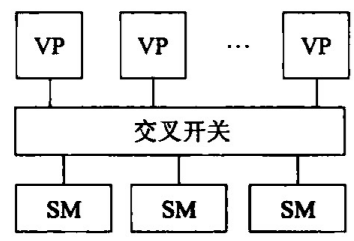• SMP, Symmetric Multiprocessor, 对称多处理机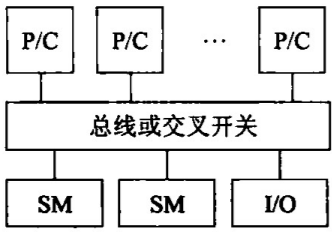• 每个处理器可同等地访问共享存储器, IO设备和操作系统服务
• 单地址空间, OS 容易调度
• 处理器不能太多
• 总线和交叉开关互连一旦做成也难以扩展
• MPP, Massively Parallel Processor, 大规模并行处理机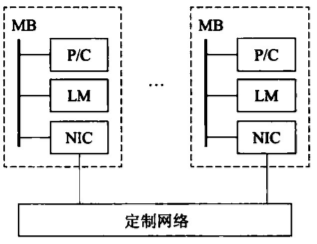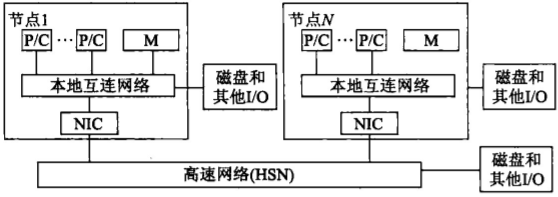• 能扩放至上万个处理器
• 程序由多个进程组成, 每个都有其私有地址空间, 进程间采用传递消息机制相互作用
• 高带宽低延迟互连网络
• DSM, Distributed Shared Memory, 分布共享存储多处理机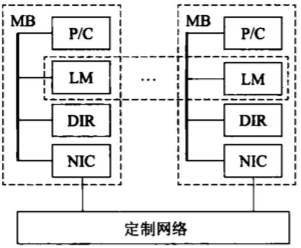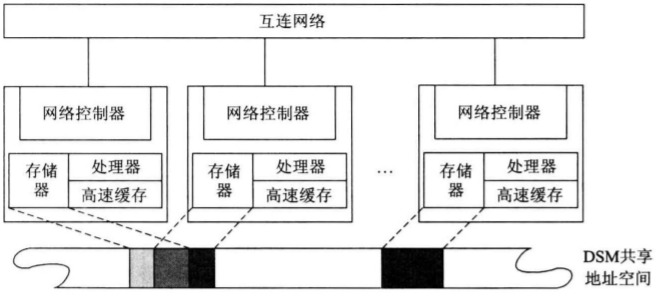• DIR 支持分布 cache 的一致性
• 与 SMP 相比, 物理上有分布在各节点中的局存, 形成共享存储器
• 单一地址编程空间, 比 MPP 编程容易
• COW, Cluster of Workstations, 工作站机群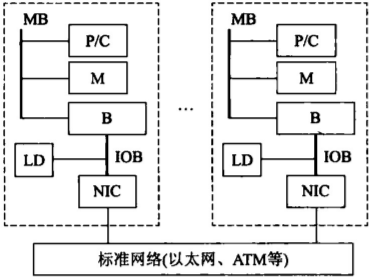• 每个节点都是一个完整的工作站 (PC, SMP)
• 标准网络 (MPP DSM 为定制网络)
• 网络接口松散 (MPP紧耦合, 网络连到节点 MB)
• 每个节点都有完整操作系统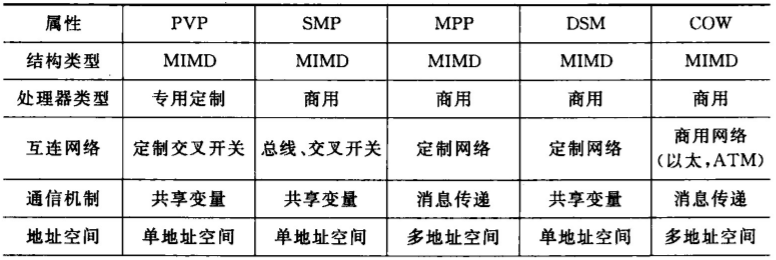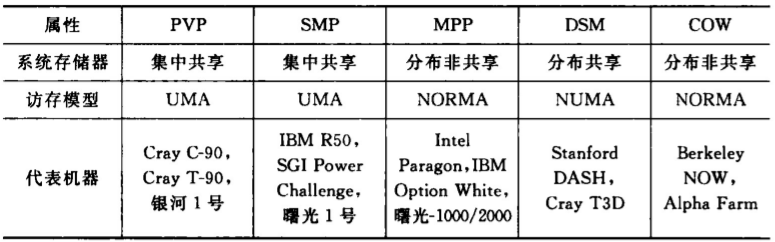• UMA, Uniform Memory Access, 均匀存储访问
• 物理存储器被所有处理器均匀共享
• 处理器访问任何存储单元的时间均相同
• 每台处理器可带私有 cache
• NUMA, NonUMA, 非均匀存储访问
• 被共享的存储器物理上分布在所有处理器中
• 所有本地存储器的集合构成全局地址空间
• 处理器访问存储单元的时间不同
• COMA, Cache Only MA, 全高速缓存存储访问
• NUMA 的一个特例
• 全部 cache 组成全局地址空间
• CC-NUMA, Coherent-Cache NUMA, 高速缓存一致性非均匀访问
• 将一些 SMP 作为单节点彼此连接
• NORMA, No-Remote MA, 非远程存储访问
• 所有存储器都是私有的, 仅由其处理器访问

## 第二章

### 静态互连网络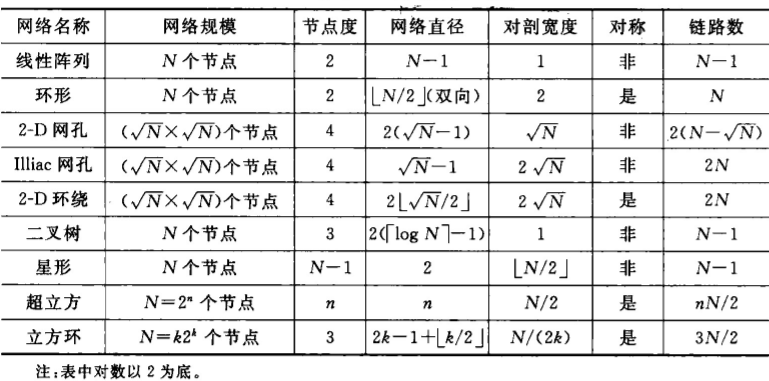### 动态互连网络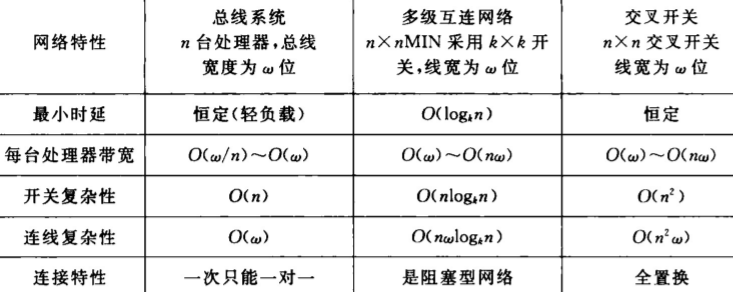## 第四章

### 加速比

$p$ 处理器数
$W = W_{series} + W_{parallel}$ 问题规模
$f = W_s / W$ 串行分量比例
$1 - f$ 并行分量比例
$T_s$ 串行执行时间
$T_p$ 并行执行时间
$S$ 加速比

#### Amdahl 定律

$S = \frac{W_s+W_p}{W_s+W_p/p} =\frac{ f + (1-f)}{f+\frac{1-f}{p}} = \frac{p}{1+f(p-1)} \rightarrow\frac{1}{f}$​

$S = \frac{W_s+W_p}{W_s+W_p/p+W_o} = \frac{p}{1+f(p-1)+W_op/W} \rightarrow\frac{1}{f+W_o/W}$

#### Gustafson 定律

$S’ = \frac{W_s+pW_p}{W_s+pW_p/p} =p-f(p-1)$

$S’ = \frac{W_s+pW_p}{W_s+pW_p/p+W_o} =\frac{f+p(1-f)}{1+W_o / W}$

#### Sun and Ni 定律

$p$ 个节点存储为 $pM$, 设 $G(p)$ 为存储增加到 $p$ 倍时负载的增加量, 则 $W = fW + (1-f)G(p)W$

$S’’ = \frac{fW + (1-f)G(p)W}{fW + (1-f)G(p)W /p} = \frac{f + (1-f)G(p)}{f + (1-f)G(p) /p}$

$S’’ =\frac{f + (1-f)G(p)}{f + (1-f)G(p) /p+W_o / W}$​

• 当 $G(p)$ = 1, 变为 Amdahl
• 当 $G(p)$​ = $p$, 变为 Gustafson

### 可扩放性

#### 等效率度量标准

$t_e^i$ 第 $i$ 个处理器的有用计算时间
$t_o^i$ 第 $i$ 个处理器的额外开销时间
$T_p = t_e^i + t_o^i = (T_e + T_o) / p$ 处理器并行算法运行时间
$W = T_e = \sum\limits_{i=0} ^{p-1} t_e^i$ 问题规模, 工作负载, 最佳串行算法所完成计算量
$S = \frac{T_e}{T_p} = \frac{p}{1+\frac{T_o}{W}}$ 加速
$E=\frac{S}{p} = \frac{1}{1+\frac{T_o}{W}}$ 效率

#### 等速度度量标准

$\Psi(1,p’) = \frac{T_1}{T_p’}=\frac{W}{W’/p’} = \frac{解决工作量为 W 的问题所需串行时间}{解决工作量为 W 的问题所需并行时间}$

#### 平均延迟度量标准

$T_i$: $p_i$ 的执行时间, 包括运行时导致的延迟 $L_i$

## 第五章

### PRAM

Parallel Random Access Machine

#### PRAM-CRCW

• 只允许同时写相同的数 CPRAM-CRCW Common
• 只允许最优先的处理器先写 PPRAM-CRCW Priority
• 允许任意处理器自由写 APRAM-CRCW Arbitrary

$T_{EREW} \ge T_{CREW} \ge T_{CRCW}$

$T_{EREW} = O (T_{CREW}) \cdot \log p = O (T_{CRCW}) \cdot \log p$

EREW 是最慢的